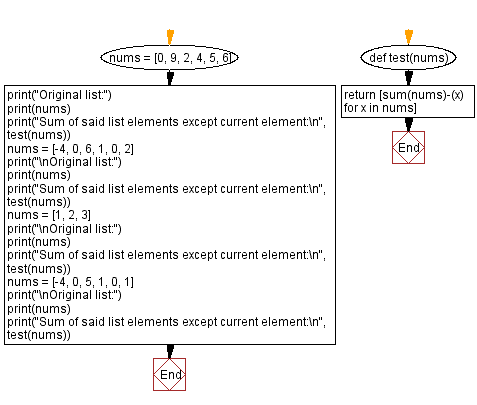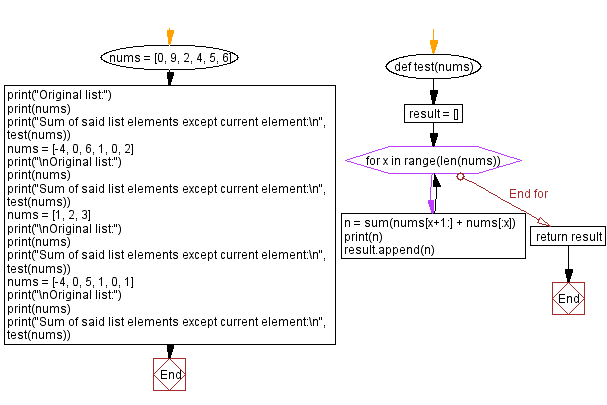﻿ Python: Sum of all list elements except current element

# Python Exercises: Sum of all list elements except current element

## Python List: Exercise - 275 with Solution

Write a Python program to add all elements of a list of integers except the number at index. Return the updated string.

Example: ([1, 2, 3]) -> [1+2+3-1, 1+2+3-2, 1+2+3-3]
-> [5, 4, 3].

Sample Data:
([0, 9, 2, 4, 5, 6] -> [26, 17, 24, 22, 21, 20]
([-4, 0, 6, 1, 0, 2]) -> [9, 5, -1, 4, 5, 3]
([1, 2, 3]) -> [5, 4, 3]
([-4, 0, 5, 1, 0, 1]) -> [7, 3, -2, 2, 3, 2]

Sample Solution-1:

Python Code:

``````def test(nums):
return [sum(nums)-(x) for x in nums]
nums = [0, 9, 2, 4, 5, 6]
print("Original list:")
print(nums)
print("Sum of said list elements except current element:\n", test(nums))
nums = [-4, 0, 6, 1, 0, 2]
print("\nOriginal list:")
print(nums)
print("Sum of said list elements except current element:\n", test(nums))
nums = [1, 2, 3]
print("\nOriginal list:")
print(nums)
print("Sum of said list elements except current element:\n", test(nums))
nums = [-4, 0, 5, 1, 0, 1]
print("\nOriginal list:")
print(nums)
print("Sum of said list elements except current element:\n", test(nums))
```
```

Sample Output:

```Original list:
[0, 9, 2, 4, 5, 6]
Sum of said list elements except current element:
[26, 17, 24, 22, 21, 20]

Original list:
[-4, 0, 6, 1, 0, 2]
Sum of said list elements except current element:
[9, 5, -1, 4, 5, 3]

Original list:
[1, 2, 3]
Sum of said list elements except current element:
[5, 4, 3]

Original list:
[-4, 0, 5, 1, 0, 1]
Sum of said list elements except current element:
[7, 3, -2, 2, 3, 2]
```

Flowchart:## Visualize Python code execution:

The following tool visualize what the computer is doing step-by-step as it executes the said program:

Sample Solution-2:

Python Code:

``````def test(nums):
result = []
for x in range(len(nums)):
n = sum(nums[x+1:] + nums[:x])
print(n)
result.append(n)
return result

nums = [0, 9, 2, 4, 5, 6]
print("Original list:")
print(nums)
print("Sum of said list elements except current element:\n", test(nums))
nums = [-4, 0, 6, 1, 0, 2]
print("\nOriginal list:")
print(nums)
print("Sum of said list elements except current element:\n", test(nums))
nums = [1, 2, 3]
print("\nOriginal list:")
print(nums)
print("Sum of said list elements except current element:\n", test(nums))
nums = [-4, 0, 5, 1, 0, 1]
print("\nOriginal list:")
print(nums)
print("Sum of said list elements except current element:\n", test(nums))
```
```

Sample Output:

```Original list:
[0, 9, 2, 4, 5, 6]
26
17
24
22
21
20
Sum of said list elements except current element:
[26, 17, 24, 22, 21, 20]

Original list:
[-4, 0, 6, 1, 0, 2]
9
5
-1
4
5
3
Sum of said list elements except current element:
[9, 5, -1, 4, 5, 3]

Original list:
[1, 2, 3]
5
4
3
Sum of said list elements except current element:
[5, 4, 3]

Original list:
[-4, 0, 5, 1, 0, 1]
7
3
-2
2
3
2
Sum of said list elements except current element:
[7, 3, -2, 2, 3, 2]
```

Flowchart:## Visualize Python code execution:

The following tool visualize what the computer is doing step-by-step as it executes the said program:

Python Code Editor:

Have another way to solve this solution? Contribute your code (and comments) through Disqus.

Previous Python Exercise: Count lowercase letters in a list of words.
Next Python Exercise: Find the largest odd number in a list of integers.

What is the difficulty level of this exercise?

Test your Programming skills with w3resource's quiz.

﻿

## Python: Tips of the Day

Unzipping:

```name = 'abcdef'
suffix = [1,2,3,4,5,6]
result = zip(name, suffix)
--> returns (a,1),(b,2),(c,3),(d,4),(e,5),(f,6)
unzipped = zip(*result)
```

We are closing our Disqus commenting system for some maintenanace issues. You may write to us at reach[at]yahoo[dot]com or visit us at Facebook